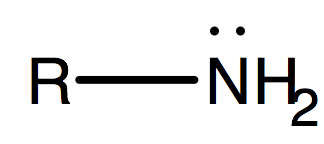# Problem: Write structural formulas for each of the following:(m) A tertiary amine with the formula C3H9N.

###### FREE Expert Solution

We’re being asked to write the structural formula for a tertiary amine with the molecular formula C3H9N.

Recall that the general structure of amines is:Also, recall that the degree of a functional group depends on the number of carbons it is directly/indirectly attached to.###### Problem Details

Write structural formulas for each of the following:

(m) A tertiary amine with the formula C3H9N.